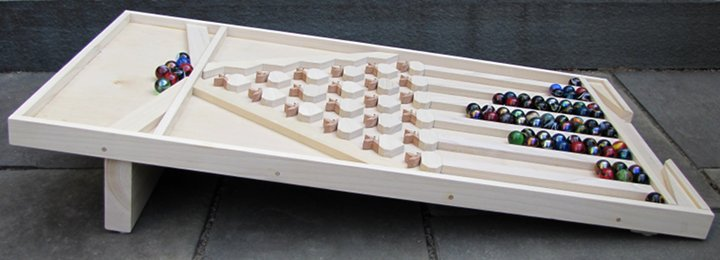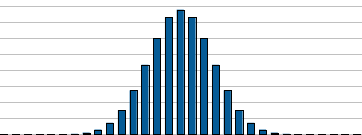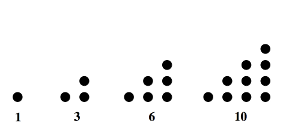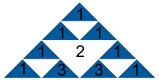Pascal's Marble Run - this example has six rows of switches and seven collecting bins.

## Pascal's Marble Run - or the Deterministic Galton Board

by Karl Sims

This marble run can be used to help understand the mathematics of probability in a physical and interactive way. Marbles rolling through a triangular array of switches and collecting in a series of bins can demonstrate concepts such as bell shaped distributions, powers of two, binary numbers, coin flipping odds, and Pascal's triangle.

Blaise Pascal was a French mathematician and inventor of mechanical calculating machines. In 1653 he described the triangle of binomial coefficients, now called Pascal's triangle, in which each number is the sum of the two above it. As you will see, elements of this triangle can be generated by this marble run.

A "Galton board" is a device in which falling balls bounce randomly left and right off of rows of pins and are collected in a number of bins at the bottom. It was invented around 1860 by Sir Francis Galton, a cousin of Charles Darwin, who used it as a tool for demonstrating the normal distribution or bell curve.

The marble run described here is a non-random variation of the Galton board that has a switch at each juncture to alternate the direction of the marbles passing through it. At each switch, if the first marble falls to the left, the next will fall to the right, and so on. This causes predictable patterns instead of random behavior, and if an even number of marbles pass through each switch, the results should exactly match the probabilities if the bounces had been random.

1. How many different paths through the switches could a marble take?   Hint   Answer

2. Tilt all the switches to the right so the first marble lands in the left-most bin. How many marbles need to roll down for one to land in the right-most bin?   Answer

3. If the switches start tilted in random directions, can you predict what state they will all be in after 64 marbles fall through?   Hint   Answer

4. You can experiment with smaller triangles by inserting marbles in the middle of the board above a specific switch instead of starting them at the top. What sequences of marble counts in the bins will you get after you insert: 4 marbles 2 switches above the bottom? 8 marbles 3 switches above the bottom? or 16 marbles 4 switches above the bottom?   Hint   Answer

5. How many possible paths lead to each switch and each bottom bin?   Hint   Answer

6. What fraction of marbles pass through each switch?   Answer

7. How often do the edge switches (the first and last in each row) get flipped?   Answer

8. If you start with all the switches tilted to the left, and somebody secretly lets some number of marbles through (between 0 and 63), can you tell how many marbles fell through just by looking at the switches?   Hint   Answer

9. For each bin at the bottom, how many times does a marble need to fall to the right to land in that bin?   Answer

10. If you flip 6 coins what are the chances they will all be heads? Note that this is the same as the chances a marble will fall left all 6 times.   Answer

11. If you flip 6 coins what are the chances that only 1 of them will be a tail? Note that this is the same as the chances a marble will fall right only once.   Hint   Answer

12. If you flip 6 coins what are the chances that you'll get 3 heads and 3 tails? Note that this is the same as the chances that a marble will take 3 lefts and 3 rights, in any order.   Hint   Answer

13. If you have 6 items how many ways can you choose 2 of them? Note that this is the same as how many ways a marble can take 2 rights.   Hint   Answer## How to build a Pascal's Marble Run

For the 6 level version shown here, you will need:

• 64 or more 1" marbles (or 15/16" or 25mm)
• 1 piece of smooth plywood for the base 19" x 36" x 1/2"
• 21 T-shaped switches 1/2" thick
• 21 bumpers 2" x 1½" x 1/2" with corners cut diagonally and 6 with bottoms cut off as shown
• 6 bin dividers 12" x 1" x 1/2"
• 4 sides 1½" x 1/2", two each of 19" and 37" lengths, one with a 7" gap for marbles to exit
• 2 removable sticks to hold back marbles 3/4" x 1/2" x 19"
• Various other guides and ramps cut from 1/2" thick wood
• Leg to keep board tilted: 19" x 5" x 1½" and optional front leg 19" x 1" x 1½" or similar
• Misc nails, tiny washers, dowels, glue, and tools

This schematic shows the dimensions and layout of the small parts. You can make the T shaped switches in two parts and glue them together, or you might be able to make them in one piece if you have tools that can machine small parts well. Drill a small hole in the center of each switch. We used a band saw with templates, drill press, and disc sander, to carefully replicate copies of each shape.

Refer to the schematic and images above for placement. Glue or nail the bumpers and guides to the board. Attach the switches with thin nails and tiny washers so they can rotate freely. Test each level to make sure the marbles can pass through the switches to the next level without getting stuck.

Note that some changes would be needed if this device were used in a public setting. The table should probably be covered so the marbles can't easily be reached, and some technique such as a conveyer belt or a pinball shooter would need to return the marbles to the top. This should also limit the rate at which the marbles are dropped to prevent them from jamming. Currently a person needs to nudge the marbles now and then to unclog them where they enter the marble run at the top.

Thanks to my sons Arlo and Felix for help building this prototype, and to the MIT Hobby Shop for the use of their tools.

## Pascal's TrianglePascal's triangle of numbers can be constructed by starting with a single 1 at the top and then growing downwards using a simple rule: each new number is the sum of the two numbers above it. On the edges we assume the empty space is 0 so the 1's are just copied down each side. Many interesting patterns and mathematical connections are hidden in Pascal's triangle...

Bell shaped curves

If you graph the values from a single row of Pascal's triangle, it forms a bell shaped curve. This "normal distribution" shows up all over the place when a number of random events are combined. Stock market returns, test scores, human heights, life spans for a given species, and so on, all tend to have bell shaped probability distributions.Graphs showing row 6 and row 30 of Pascal's triangle (with different scales)

Binomial coefficients

Pascal's triangle also provides a quick way to look up binomial coefficients. For example, to expand (x+y)6 the number of each x and y power combination follows row 6 of Pascal's triangle:
(x+y)6 = 1 x6 + 6 x5 y + 15 x4 y2 + 20 x3 y3 + 15 x2 y4 + 6 x y5 + 1 y6

Powers of 2

Sum each row of Pascal's triangle to get the powers of two: 1, 2, 4, 8, 16, 32, 64...

Powers of 11

If you collapse each row into a single number by taking each element as a digit (and carrying over to the left if the element has more than one digit) you get the powers of eleven: 1, 11, 121, 1331, 14641, 161051...Added adjacent triangulars give squares
Square numbers

The 3rd diagonal contains the "triangular numbers" (1, 3, 6, 10, 15, 21, 28, 36, 45...) and if you add adjacent pairs of these, you get the perfect squares: 1+3=4   3+6=9   6+10=16   10+15=25   and so on.

The Choose function

Say you have 6 items and you want to know how many different ways you can choose 2 of them. The answer of "6 choose 2" is 6 x 5 / 2 = 15. There are 6 ways to choose the 1st item and then 5 left to chose the 2nd item from, but that includes both orderings of the 2 items so we divide by 2 since the order doesn't matter. The general equation for "N choose K" is N! / (N-K)! / K! and this formula can be used to calculate an arbitrary binomial coefficient or element of Pascal's triangle. Row N number K (starting at 0) in Pascal's triangle equals "N choose K".

In the "12 Days of Christmas" song, the number of gifts can be found in Pascal's triangle. The 3rd diagonal contains the "triangular numbers" which are each the sum of the first N integers. The 4th diagonal contains the "tetrahedral numbers" (1, 4, 10, 20, 35, 56, 84, 120...) which are each the sum of the first N triangular numbers. If you stack triangles of sizes 1 to N you get a 3D tetrahedron with N per edge. In the classic Christmas song "my true love gave to me" N new gifts each day plus all the gifts from previous days are repeated, so the number of gifts received on each day are the triangular numbers, and the cumulative total gifts are the tetrahedral numbers. The grand total gift count is the 12th tetrahedral number: 364.Triangular numbers Tetrahedral numbers

If N people are at a party and all click their glasses with each other, how many total clinks are there? Each person clinks N-1 others, so avoiding double counting, the answer is N(N-1)/2. This is also "N choose 2" and is also the sum of 1 to N-1. It is also the Nth triangular number found in the 3rd diagonal of Pascal's triangle.

Prime numbersRows with prime multiplesFibonacci numbers
If the 2nd number in a given row is a prime number, all the numbers in that row, except the 1's, are multiples of that same prime number. All the elements in the downward pointing triangle from this row are also multiples of that same prime, since when you add two multiples of the same number you get another multiple of that number. It also appears that if the 2nd number in a given row is any power of a prime number, all the numbers in that row except the 1's are multiples of that prime number.

Fibonacci numbers

If you sum the numbers in each skewed diagonal you get the Fibonacci sequence: 1, 1, 2, 3, 5, 8, 13, 21, 34... Each new Fibonacci number is just the sum of the previous two.

Sums of Squares

The sum of the squares of any row of Pascal's triangle equals the center element in the row twice as far down. In other words if you draw a downward pointing triangle so the top edge includes an entire row, the element at the bottom tip of that triangle will equal the sum of the squares of that top row. For example: 12 + 22 + 12 = 6   or 12 + 32 + 32 + 12 = 20.Sierpinski's triangle fractal

Print out a large Pascal's triangle and see what kind of pattern you get when you draw a colored triangle or circle over each odd number. Can you explain why this pattern occurs? Which rows are all odd? For the impatient, here is the resulting pattern. For variations on this, try instead coloring in all the multiples of 3, or all the multiples of 5. Multiples of prime numbers seem to give nice regular patterns, where non-primes are less regular.